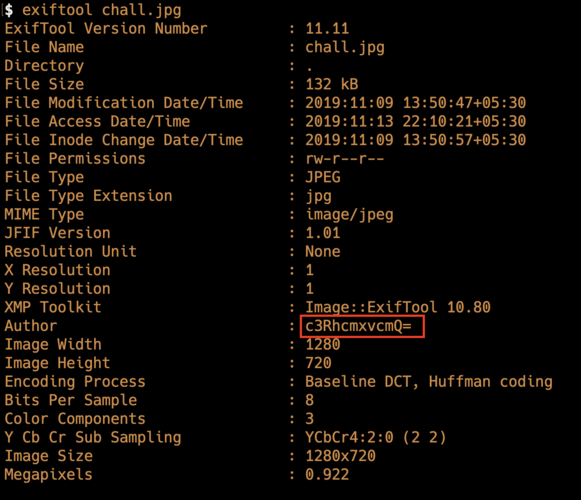# inCTF 2019 writeup

inCTF is jeopardy style ctf challenge which is conducted by Amritha university. The event is hosted in online and i participated.

I manage to solve two challenges

### Easy Peasy

The Easy Peasy challenge we got a image file, when i check the authorit is a base64 encoded so i decoded the string we get ( for decoding you can use cyberchef)

starlord i guess it must be password so i searched for stegnography decoding with password i found this https://futureboy.us/stegano/decinput.html

if you don’t know what stegnography is,it is way to hiding information inside a file.

upload the given image and used the password starlord got the flag

``inctf{h1d1ng_d474_1n_0th3r_f1le5_1s_r34lly_c00l!!!!!}``

### Python_revN

They have given the source code we need to reverse engineer it.

``````import string
import random

def push(x):

upper = string.ascii_uppercase
lower = string.ascii_lowercase
digits = string.digits
n = []
a = ""
for i in x:
if i.isupper() is True:
n.append(upper[(upper.index(i)+3)%26])
elif i.islower() is True:
n.append(lower[(lower.index(i)+3)%26])
elif i.isdigit() is True:
n.append(digits[(digits.index(i)+3)%10])

for j in n:
a+=j

return a

def sage(x):
final=[]
for i in range(0,len(x),4):
temp=x[i:i+4].encode('utf-8').hex()
k=int(temp,16)
final.append(k)

return final

if **name**=="**main**":
print("Get the flag if you can!")
x = input().encode('utf-8').hex()
check = [959592808, 959852599, 960049253, 926430775, 892811314, 946419251, 929576502, 946419765, 909391664, 925972535, 892613940, 912864564, 12391]
ALL = []
ALL=sage(push(x))
count = 0

for i in range(len(ALL)):
if check[i] == ALL[i]:
count+=1
if count == 13:
print("Go Kid Submit The Flag")
else:
print("Wrong Turn!")
``````

Inorder to understand this problem i used pen and paper and passed a easy input so i can able to understand what is happening in each function

i gave AA as input i/p: AA

step 1: Hex encoding the input 4141 step 2: It is passed to push function where it will iterate over the above input 4141 three case: To make it easy to understand i seperated the logic into three statements

• If the character is Upper case
``````index <= upper.index(i) + 3
newIndex <= index % 26
get <= upper[newIndex]``````
• If the character is Lower case
``````index <= lower.index(i) + 3
newIndex <= index % 26
get <= lower[newIndex]``````
• If the character is Upper case
``````index <= digits.index(i) + 3
newIndex <= index % 10
get <= digits[newIndex]``````

combine all the character which is generated from above into get variable, so now 4141 will be changed to 7474

step 3: sage function the given input is seperated into four bits and encoded into hex then the hex is converted into int using int(,16) which will give as 926168884 the above value get append to final array which is returned.

### Decrypting the Program

Now we are going do the above thing in reverse to retrive the string

``````
import string
import random

upper = string.ascii_uppercase
lower = string.ascii_lowercase
digits = string.digits
final_string = ""

# data = 959592808
check = [959592808, 959852599, 960049253, 926430775, 892811314, 946419251, 929576502, 946419765, 909391664, 925972535, 892613940, 912864564, 12391]

for data in check:

# convert the int to hex ( step 3 )

hexlify=format(data,'x')
print("Hex formated data "+hexlify)

convert_bytes = bytes.fromhex(hexlify).decode()
print("The decoded value is"+convert_bytes)

# decode hex into bytes

n = []
a = ""

# Reversing the push function (step 2)

for i in convert_bytes:
if i.isupper is True:
n.append(upper[(upper.index(i)-3)%26])
elif i.islower() is True:
n.append(lower[(lower.index(i)-3)%26])
elif i.isdigit() is True:
n.append(digits[(digits.index(i)-3)%10])

for j in n:
a+=j

# Decoding hex (step 1)

decoded = bytes.fromhex(a).decode()

final_string+=decoded
print("Decoded: "+decoded)

print("Flag: "+final_string)
``````

combine all the decoded data into final_string and print it. you will get the flag

``inctf{E4\$Y_0N3_R1GHT!!?!}``